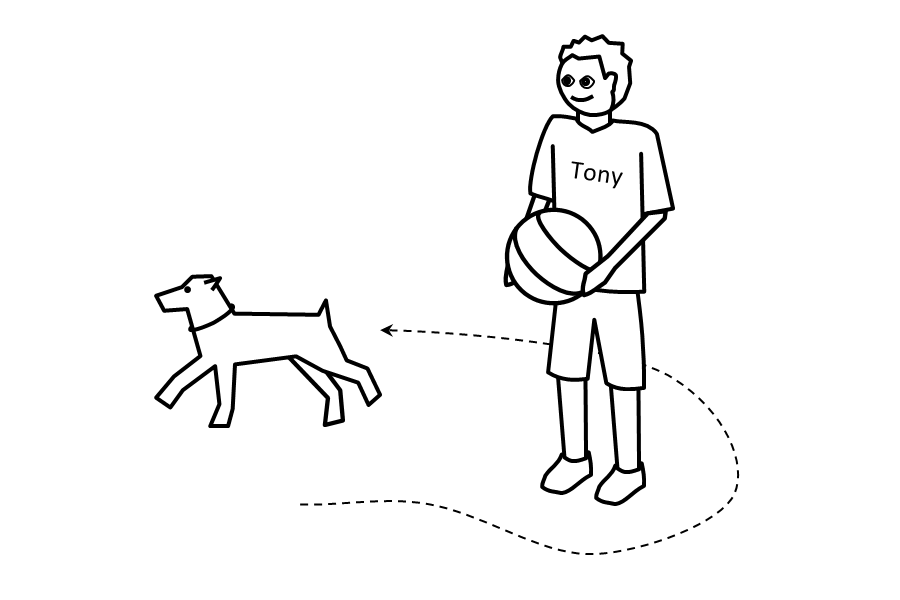## Learn These Words First (6D)

6-13. quick, quickly.

[J causes K to happen quickly.] = Often someone does something for a long time to cause something like K to happen. But J does something for a short time that causes K to happen.

[The animal was here a short time before now, but now it is far from here because it moved quickly.]

6-14. centre, (center), centres, (centers), centre of, centres of.

[J is the centre of K.] = J is a small place between one side of K and the other side of K, like this: The distance between J and one side of K is the same as the distance between J and the other side of K. The distance between J and the front of K is the same as the distance between J and the back of K. The distance between J and the top of K is the same as the distance between J and the bottom of K.

[The people moved towards the centre of the building.]

6-15. round.

[X is round.] = You think like this about the shape of X: The distance between the centre of X and each surface part of X is the same as the distance between the centre of X and each other surface part of X.

[This kind of plant has round parts that you can eat.]

6-16. around.

[J is around K.] = J is near the front and back and sides of K. J is near K like something long that has parts near K on all sides.

[There is water around me on all sides.]

[J moves around K.] = J moves like this: From a place in front of K, J moves to one side of K, then J moves behind K, then J moves to the other side of K, and then J moves to the front of K.

[The animal moved around the building.]Tony is holding something round.

The small animal __________.